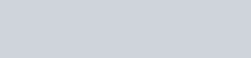# Swift Pattern Matching – if case, for case, switch case

Filed Under: Swift

In this tutorial, we’ll look into Pattern Matching in Swift. Pattern matching is seen in switch statements.

## Swift Pattern Matching

The easy to use switch syntax of Swift can be extended to for and if statements as well.
Pattern matching is used to match tuples, arrays, enums etc. or a part of them.

Here’s a very basic example of Pattern matching:

``````
for _ in 1...10{
}
``````

Let’s fire up our XCode Playground and start Swifting.

### Partial Matching Tuples

In Switch statements, partial matching is very commonly used and implemented as shown below:

``````
let author1 = ("Anupam","Android")
let author2 = ("Pankaj","Java")
let author3 = ("Anupam","Swift")
let author4 = ("Anupam","Java")

func switchCasePatternTuple(tuple : (String,String))
{

switch tuple {
case (let name, "Java"):
print("\(name) writes on Java")

case let(_,category):
print("Some author writes on \(category)")

}
}

switchCasePatternTuple(tuple: author1)
switchCasePatternTuple(tuple: author2)
switchCasePatternTuple(tuple: author3)
switchCasePatternTuple(tuple: author4)
``````

In the above code, we are partially matching only one of the values in the tuple.
Following is the output:Swift Pattern Matching Tuple Partial Output

We can write let to each parameter name or like case let as we did in the above snippet.
`let` is used to bind associated values.

### Matching Optionals

Swift has a way of matching optionals.
We can do that by using the `.some` and `.none` syntax or just setting a ? on the parameter.

``````
let y : Int? = 1
if case .some = y{
print("Value of optional is \(y)")
}

let x : Int? = 5
if case .some = x{
print("Value of optional is \(x)")
}

if case .none = x
{
print("Optional x is nil")
}

if case let z? = x, z>0{
print("Value of implicitly unwrapped optional is \(z)")
}
``````

`if case let x = y` is equal to `switch y {case let x: }`

The output printed in the console is:

``````
Value of optional is Optional(1)
Value of optional is Optional(5)
Value of implicitly unwrapped optional is 5
``````

Let’s look at a switch case example for optional matching:

``````
let name : String? = "Anupam"
let password : String? = "ABCD"

switch userInfo {
case let (.some(name), nil):
print("\(name) does not remember the password.")
case (.none, .some(_)):
print("There is some password but no name.")
case (.none, _):
print("No user name. No password. ")
}

``````

Note: if name and password both are nil, the fourth case would be executed.

Also, instead of some and none, we can use the following syntax as well:

``````
switch userInfo {
case let (name?, nil):
print("\(name) does not remember the password.")
case (.none, _?):
print("There is some password but no name.")
case (.none, _):
print("No user name. No password. ")
}
``````

Let’s look at the for case to match optionals.

``````
let topics =  ["Java","Android","Python","Django","JS",nil]

for case let .some(t) in topics
{
print("Topic is \(t)")
}

for case let .some(t) in topics where t.starts(with: "J")
{
print("Topic starting with J is \(t)")
}
``````Swift Pattern Matching Optionals Output

where clause is used to set a pattern matching on the optional case.

### Matching Types

We can match types in the switch statements as shown below:

``````

var randomArray: [Any] = ["Swift", 2, 7.5]
for randomItem in randomArray {
switch randomItem {
case is Int:
print("Int value. I don't care about the value")
case is String:
print("String type.")
default: break
}
}
``````

### ~= operator

We can use the ~= operator in the if statements to match a range as shown below:

``````
let age = 25

if 0..<13 ~= age{
print("Hey kid!!")
}
else if 13...19 ~= age{
print("Hey teenager!!")
}
else if 19...45 ~= age{
}

``````

The ~= operator can be replaced by = or .contains(age) as well but that would cause readability issues.

### Pattern Matching With Enums

The last example deals with Pattern matching using Swift Enums.

``````
enum Month{
case Jan(zodiac: String,gender:String)
case Feb
case March(zodiac: String)
}

let month1 = Month.March(zodiac: "Pisces")
let month2 = Month.March(zodiac: "Aries")
let month3 = Month.Jan(zodiac: "Aqarius", gender: "Female")

func switchEnum(month: Month)
{
switch month {
case .Feb:
print("Feb it is")
case let .March(zodiac) where zodiac == "Pisces":
print("March Pisces. Aries won't fall here.")
case let .Jan(_,gender):
print("Jan does not care about zodiac. Only shows gender \(gender)")

default:
print("Others")
}
}

switchEnum(month: month1)
switchEnum(month: month2)
switchEnum(month: month3)

//prints:
//March Pisces. Aries won't fall here.
//Others
//Jan does not care about zodiac. Only shows gender Female
``````

This brings an end to this tutorial on Pattern Matching in Swift.

1.Mahitha says:

I want to know below concepts:
1. method columns
2. static methods
3. instant methods
4. object class methods